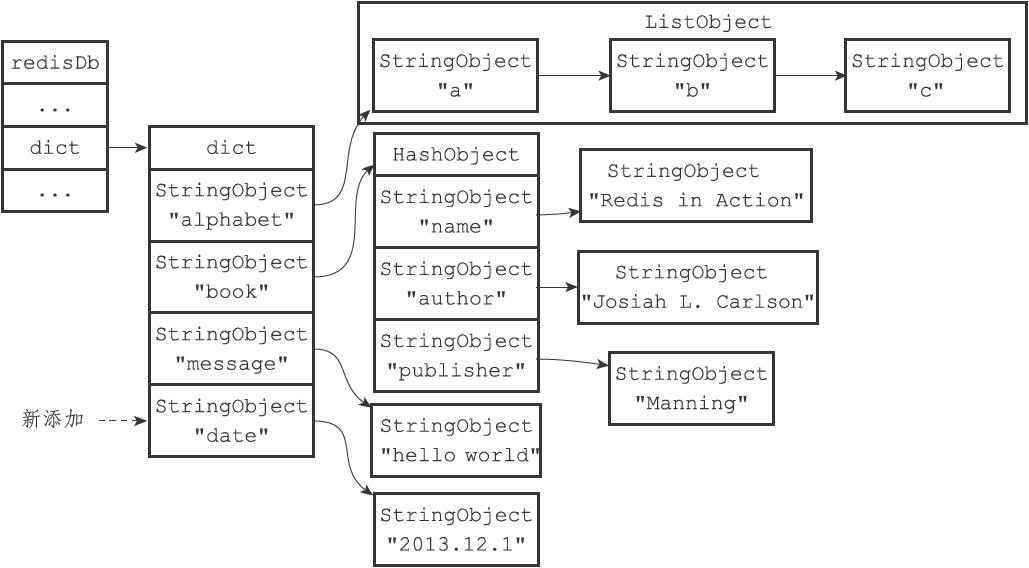# sds.h sds.c

## 内存对齐

__attribute__((__packed__))可以让编译器对结构体不进行内存对齐，详细参考

## 宏##

##后标识的字符串会被替换，然后其左右的内容加上自己会被合并到一起，编译器将其视为标识符进行解析，详细参考

## sds.h 源码

sds 可以被简单的认为是一个 char*

__attribute__ ((__packed__))是编译器指令，可以取消内存对齐，让内存紧凑排列，这部分首先看后四个结构体，他们的内存结构定义几乎一摸一样。

• len: 字符串的长度

• alloc： 分配的空间大小

• flags： 字符串的类型（5种），所以只有最低的三位有意义，高5位不做使用。

• buf： 字符串的实际内容

sds 把字符串的内容，以及他的元信息（字符串类型、字符串长度、字符串分配的空间）储存在了一起，让内存排列更加紧致。

# mt19937-64.c mt19937-64.h

## 线性反馈移位寄存器

• 寄存器输出最低位x（x=0或1）。
• 寄存器选择被标记的位和x，取出其值，放到一起进行异或，得到y（y=0或1）。
• 寄存器把自己右移1位，然后把值y放入最高位。

……

## 梅森旋转算法

$32$ 位的梅森旋转算法能够产生周期为 $P$ 的 $w$-比特的随机数序列${\vec x_i}$；其中 $w=32$。这也就是说，每一个$\vec x$ 是一个长度为 $32$ 的行向量，并且其中的每一个元素都是二元数域$\mathbb{F}_2 \overset{\text{def}}{=} {0, 1}$中的元素。现在，我们定义如下一些记号，来描述梅森旋转算法是如何进行旋转（线性移位）的。

• $n$：参与梅森旋转的随机数个数；
• $r$：$[0, w)$ 之间的整数；
• $m$：$(0, n]$之间的整数；
• $\mathbf{A}$：$w \times w$ 的常矩阵；
• $\vec x^{(u)}$：$\vec x$的最高 $w - r$ 比特组成的数（低位补零）；
• $\vec x^{(l)}$：$\vec x$的最低 r 比特组成的数（高位补零）。

$$\vec x_0, \vec x_1, \ldots, \vec x_{n - 1}.$$

$$\begin{equation}\vec x_{k + n} \overset{\text{def}}{=} \vec x_{k + m}\oplus \bigl(\vec x_{k}^{(u)}\mid \vec x_{k + 1}^{(l)}\bigr)\mathbf{A}.\label{eq:twister}\end{equation}$$

$$\begin{pmatrix} & 1 \ & & 1 \ & & & \ddots \ & & & & 1 \ a_{w - 1} & a_{w - 2} & a_{w - 3} & \cdots & a_0 \end{pmatrix}$$# dict.c dict.h

## 字典源码

redis是单线程，绝对不能出现过于耗时的操作，否则影响redis延时

# intset.c intset.h

encoding是编码方式，指的是contents中的数据如何储存，编码方式分为三种

length是数字的个数

contents是内容，但是他不一定是8位的整数，取决于encoding的值。

redis对象都在这里统一起来

# server.h-3-db# rio.c rio.h

rio即redis io， 主要实现了redis中的io操作, rio是一个结构体，他就是_rio, 下面是源码。

write 写数据，是函数指针
tell tell，是函数指针
flush flush，是函数指针
update_cksum 校验和，是函数指针
cksum 当前校验和
flags 是否发生读写错误
processed_bytes 已经处理的字节数
max_processing_chunk 单次最大处理的字节数
io 具体的读写目标

redis对多个操作系统做了兼容,在linux下redis_fsync就是fsync，文件读写也有自己的缓冲区，一旦开启了自动同步io.file.autosync，则每写入一定数量io.file.buffered的数据，就进行同步fsync(fileno(fp))

# rdb.c rdb.h

## rdbSaveBackground

fork出一个子进程，子进程执行rdb任务。